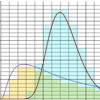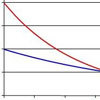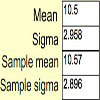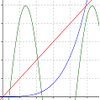# Resources tagged with: Probability distributions, expectation and variance

Filter by: Content type:
Age range:
Challenge level:

There are 16 NRICH Mathematical resources connected to Probability distributions, expectation and variance, you may find related items under Advanced Probability and Statistics.

Broad Topics > Advanced Probability and Statistics > Probability distributions, expectation and variance### Aim High

##### Age 16 to 18 Challenge Level:

How do you choose your planting levels to minimise the total loss at harvest time?##### Age 16 to 18 Challenge Level:

Can you work out the means of these distributions using numerical methods?### Time to Evolve 2

##### Age 16 to 18 Challenge Level:

How is the length of time between the birth of an animal and the birth of its great great ... great grandparent distributed?### Stats Statements

##### Age 16 to 18 Challenge Level:

Are these statistical statements sometimes, always or never true? Or it is impossible to say?### Into the Exponential Distribution

##### Age 16 to 18 Challenge Level:

Get into the exponential distribution through an exploration of its pdf.### Random Inequalities

##### Age 16 to 18 Challenge Level:

Can you build a distribution with the maximum theoretical spread?### Spinners

##### Age 16 to 18 Challenge Level:

How do scores on dice and factors of polynomials relate to each other?### A Well-stirred Sample

##### Age 16 to 18 Challenge Level:

Typical survey sample sizes are about 1000 people. Why is this?### Binomial or Not?

##### Age 16 to 18 Challenge Level:

Are these scenarios described by the binomial distribution?### What Is a Random Variable, Really?

##### Age 16 to 18### Binomial Conditions

##### Age 16 to 18 Challenge Level:

When is an experiment described by the binomial distribution? Why do we need both the condition about independence and the one about constant probability?### Can You Find ... Random Variable Edition

##### Age 16 to 18 Challenge Level:

Can you create random variables satisfying certain conditions?### Statistics - Maths of Real Life

##### Age 14 to 18 Challenge Level:

This pilot collection of resources is designed to introduce key statistical ideas and help students to deepen their understanding.### Exponential Intersection

##### Age 16 to 18 Challenge Level:

Can the pdfs and cdfs of an exponential distribution intersect?### Understanding Hypotheses

##### Age 14 to 18